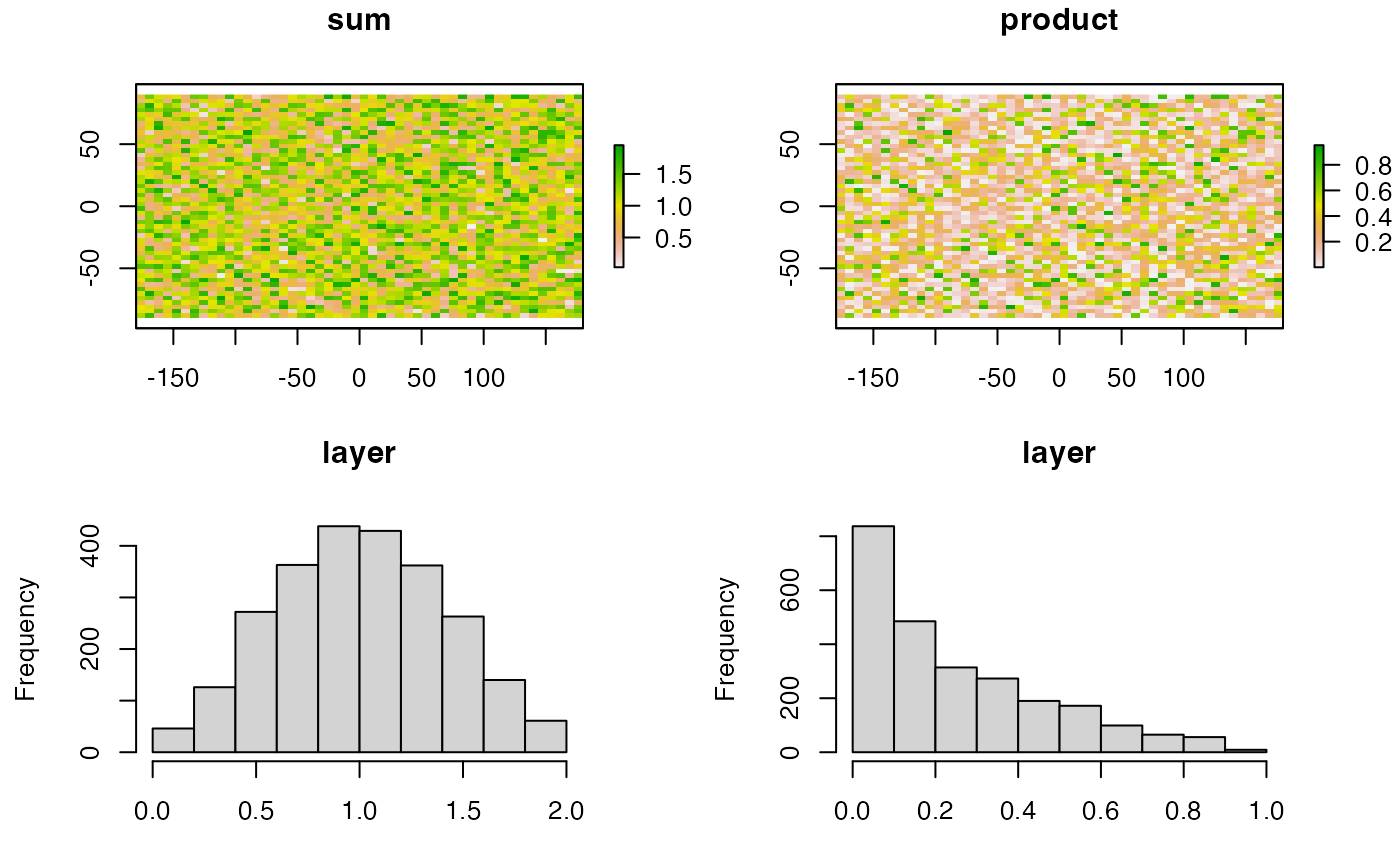Create a histogram of the values of a RasterLayer. For large datasets a sample is used.

# S4 method for Raster
hist(x, layer, maxpixels=100000, plot=TRUE, main, ...)

## Arguments

x

Raster* object

layer

integer (or character) to indicate layer number (or name). Can be used to subset the layers to plot in a multilayer Raster* object

maxpixels

integer. To regularly subsample very large objects

plot

logical. Plot the histogram or only return the histogram values

main

character. Main title(s) for the plot. Default is the value of names

...

Additional arguments. See under Methods and at hist

## Value

This function is principally used for the side-effect of plotting a histogram, but it also returns an S3 object of class 'histogram' (invisibly if plot=TRUE).

pairs, boxplot

## Examples

r1 <- raster(nrows=50, ncols=50)
r1 <- setValues(r1, runif(ncell(r1)))
r2 <- setValues(r1, runif(ncell(r1)))
rs <- r1 + r2
rp <- r1 * r2
par(mfrow=c(2,2))
plot(rs, main='sum')
plot(rp, main='product')
hist(rs)
a = hist(rp)a
#> $breaks #>  0.0 0.1 0.2 0.3 0.4 0.5 0.6 0.7 0.8 0.9 1.0 #> #>$counts
#>   837 485 314 273 190 172  99  65  56   9
#>
#> $density #>  3.348 1.940 1.256 1.092 0.760 0.688 0.396 0.260 0.224 0.036 #> #>$mids
#>   0.05 0.15 0.25 0.35 0.45 0.55 0.65 0.75 0.85 0.95
#>
#> $xname #>  "v" #> #>$equidist
#>  TRUE
#>
#> attr(,"class")
#>  "histogram"